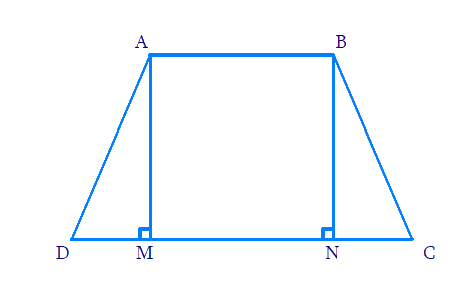In the verge of coronavirus pandemic, we are providing FREE access to our entire Online Curriculum to ensure Learning Doesn't STOP!

# Ex.10.5 Q8 Circles Solution - NCERT Maths Class 9

Go back to  'Ex.10.5'

## Question

If the non-parallel sides of a trapezium are equal, prove that it is cyclic.

Video Solution
Circles
Ex 10.5 | Question 8

## Text Solution

What is known?

Non-parallel sides of trapezium are equal.

What is unknown?

To prove that trapezium is cyclic.

Reasoning:

If the sum of a pair of opposite angles of a quadrilateral is $$180^{\circ},$$ the quadrilateral iscyclic. Using Right angled-Hypotenuse-Side (RHS) criteria and Corresponding parts of congruent triangles (CPCT) we prove the statement.

Steps:

Draw a trapezium $${ABCD}$$

with $${AB} \| {CD}$$

$$AD$$ and $$BC$$ are the non-parallel sides which are equal. $${AD = BC.}$$

Draw \begin {align} {AM} \perp {CD} \end {align}

and \begin {align} {BN} \perp {CD} \end {align}Consider $$\Delta {AMD}$$ and $$\Delta \text{BNC.}$$

\begin{align} {AD} &={BC} \qquad \; (\text { Given }) \\\angle{AMD}&=\angle {BNC} \quad \left(90^{\circ}\right) \end{align}

$$AM =BN$$ (Perpendicular distance between two parallel lines is same)

By RHS congruence,

\begin {align}\Delta {AMD} \cong \Delta {BNC} \end {align}

Using CPCT ,

\begin {align} \angle {ADC}=\angle {BCD} \ldots . .(1) \end {align}

$$\angle {BAD}$$ and $$\angle {ADC}$$ are on the same side of transversal $$\angle \text{AD}.$$

\begin{align}\angle {BAD}+\angle {ADC}&=180^{\circ} \\ \angle {BAD}+\angle {BCD}&=180^{\circ} \text{[Using}(1)]\end{align}

This equation proves that the opposite angles are supplementary.

Hence, $${ABCD}$$ is a cyclic quadrilateral.

Video Solution
Circles
Ex 10.5 | Question 8

Learn from the best math teachers and top your exams

• Live one on one classroom and doubt clearing
• Practice worksheets in and after class for conceptual clarity
• Personalized curriculum to keep up with school